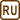## Work Performed by a Steam Engine

### Task number: 1803

The temperature of steam coming from a boiler to a cylinder of a steam engine is 120 °C; the steam condenses in a cold reservoir with a temperature of 40 °C. What is the maximum work performed by the engine in ideal conditions with a heat consumption of 4.2 kJ?

• #### Hint 1

If we want the efficiency to be maximal, the engine must work according to Carnot cycle.

• #### Hint 2

Find the definition of an efficiency of a heat engine in general, and the definition of Carnot engine efficiency.

• #### Analysis

First we write down the efficiency of a heat engine as the performed work divided by the supplied heat.

The efficiency of the engine is maximal provided that the engine operates according to the Carnot cycle. In such a case it would perform maximum work with the same heat supplied. The efficiency of Carnot cycle depends only on the temperatures between which it operates.

By comparing both terms we obtain the expression for the efficiency, from which we evaluate the performed work.

• #### Given values

 t1 = 120 °C => T1 = 393 K temperature of steam (hot reservoir) t2 = 40 °C => T2 = 313 K temperature of cold reservoir Q = 4.2 kJ = 4.2·103 J heat consumption W = ? maximum work performed by engine
• #### Solution

The efficiency η of a heat engine is defined as the ratio of performed work W and supplied heat Q. Therefore it applies

$\eta=\frac{W}{Q}.$

Maximum efficiency is achieved when the engine operates according to Carnot cycle; the efficiency η depends only on the temperature T1 of the hot reservoir and the temperature T2 of the cold reservoir. Therefore the following applies

$\eta=\frac{T_1-T_2}{T_1}.$

In our case, the temperature of the hot reservoir corresponds to the temperature of the steam in the cylinder of the steam engine.

By comparing the two equations for efficiency we obtain an equation

$\frac{W}{Q}=\frac{T_1-T_2}{T_1}.$

We evaluate the work performed by the engine from this equation:

$W=\frac{Q\left(T_1-T_2\right)}{T_1}.$
• #### Numerical solution

$W=\frac{Q\left(T_1-T_2\right)}{T_1}= \frac{4.2\cdot{ 10^3}\cdot \left(393-3135\right)}{393}\,\mathrm{J}\,\dot{=}\,850\,\mathrm{J}$
• #### Answer

Maximum work performed by the engine is approximately 850 J.